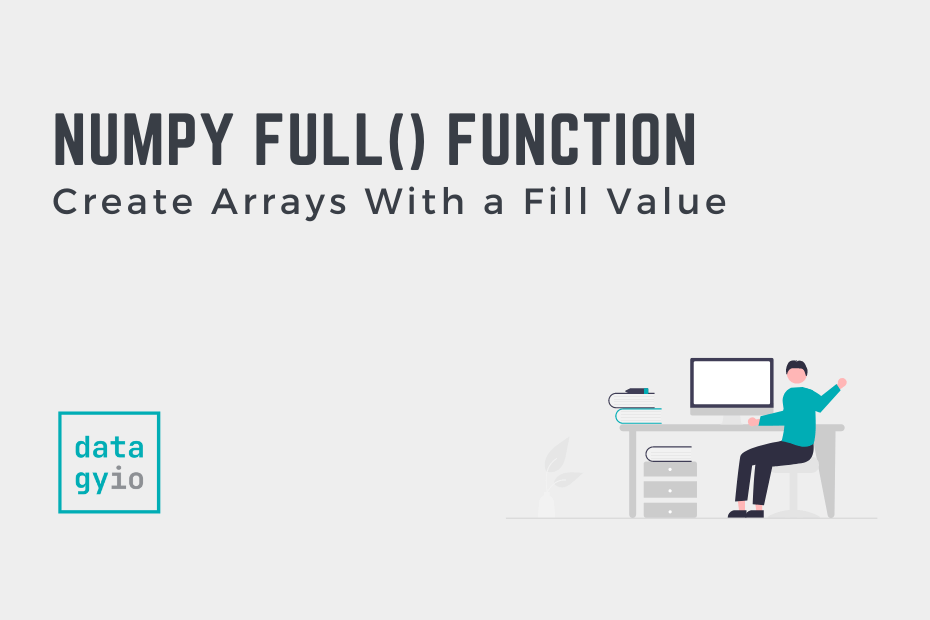# NumPy full: Create Arrays With a Fill ValueNumPy arrays are essential to most data analysis and data science workflows in Python. Because of this, being able to generate arrays is an important skill. In this tutorial, you’ll learn how to use the NumPy full() function to generate arrays filled with a given value. This function is similar to the NumPy zeroes() and NumPy ones() functions, though it generates arrays of different shapes filled with a given number.

By the end of this tutorial, you’ll have learned:

• How the NumPy full() function works
• How to generate one-dimensional and two-dimensional arrays filled with a number
• How to set the data type when generating arrays with NumPy full()

## Understanding the NumPy full() Function

What is the NumPy full() function?

The NumPy full() function is used to generate arrays filled with a given number. The function can generate one-dimensional and two-dimensional arrays of a specified data type.

Before diving into using the NumPy `full()` function, let’s explore how the function is made up. This allows you to better understand the parameters and default arguments of the function. Knowing this prepares you to understand how to customize the output of the resulting function.

``````# Understanding the NumPy full() Function
import numpy as np
arr = np.full(shape, fill_value, dtype=None, order='C')``````

We can see that the function has four different parameters, two of which have default arguments provided. The table below breaks down these arguments in detail:

Now that you have a strong understanding of the function, let’s dive into how we can use the function to generate arrays.

## Generate a 1-D NumPy Array Filled With the Same Number

NumPy makes it very easy to create one-dimensional arrays filled with a given value. This can be done by passing a single integer into the `shape=` parameter. This allows you to specify the length of the resulting array. By passing a second value into the function, you can specify the value you want to fill the array with.

Let’s generate an array with five values, containing the value 3:

``````# Generate 1-D Arrays with np.full()
import numpy as np
arr = np.full(shape=5, fill_value=3)
print(arr)

# Returns: [3 3 3 3 3]``````

In the code block above, we pass the values of 5 and 3 into the `full()` function. Because the `shape=` and `fill_value=` parameters are the first and second values positionally, we can avoid using their keywords.

## Generate a 2-D NumPy Array Filled With the Same Number

To generate a two-dimensional NumPy array filled with a given value, you can pass a tuple of values into the shape parameter. This allows you to set the dimensions of the resulting array. Let’s see how we can create a 3 by 3 array containing the value 3.

``````# Generate 2-D Arrays with np.full()
import numpy as np
arr = np.full(shape=(3, 3), fill_value=3)
print(arr)

# Returns:
# [[3 3 3]
#  [3 3 3]
#  [3 3 3]]``````

Let’s break down what we did above:

1. We passed in `(3, 3)` to declare that we want a 3 by 3 array
2. We also passed in the fill value of 3, to fill the array with the value of 3

In the following section, you’ll learn how to specify the data type when generating NumPy arrays.

## Modify the Data Type When Generating NumPy Arrays with full()

By default, the NumPy `full()` function will use the data type of the fill value that was passed in. This means that, in the previous examples, the data type of the value was an integer. However, we can specify the data type of the elements in the array by using the `dtype=` parameter. Let’s see how we can recreate the array from above, using floating point values.

``````# Modify the Data Type of Arrays Generated with np.full()
import numpy as np
arr = np.full(shape=(3, 3), fill_value=3, dtype='float')
print(arr)

# Returns:
# [[3. 3. 3.]
#  [3. 3. 3.]
#  [3. 3. 3.]]``````

By passing in `'float'` as the third argument, we can specify the data type of the resulting array to be of floats. This is despite passing in an integer as the fill value argument.

## Conclusion

In this tutorial, you learned how to generate NumPy arrays of a given fill value using the `np.full()` function. You first learned how the function is developed by gaining an understanding of the parameters and default arguments of the function. Then, you learned how to generate one-dimensional and two-dimensional arrays with a fill value. Finally, you learned how to specify the data type of the resulting array.